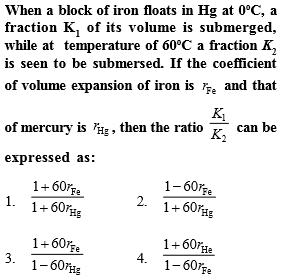# NEET Physics Thermal Properties of Matter Questions Solved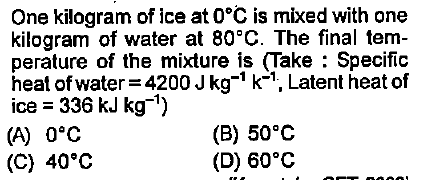Complete Question Bank + Test Series
Complete Question Bank

Difficulty Level:

1.  15 g

2.  30 g

3.  50 g

4  60 g

Complete Question Bank + Test Series
Complete Question Bank

Difficulty Level:

The fastest mode of heat transfer is

1.  Conduction

3.  Convection

4.  all are  equally fast

Complete Question Bank + Test Series
Complete Question Bank

Difficulty Level:

1.  310.9

2.  37.8

3.  100

4.  122.4

Complete Question Bank + Test Series
Complete Question Bank

Difficulty Level:

A black body at temperature 300K radiates heat at the rate E. If its temperature is increased by 600k, the rate of radiation will increase to

1.  16E

2.  64E

3.  81E

4.  256E

Complete Question Bank + Test Series
Complete Question Bank

Difficulty Level:

1.  Less than 5 minutes

2.  equal to 5 minutes

3.  More than 5 minutes

4.  Can't say anything like the temperature of surrounding is not known

Complete Question Bank + Test Series
Complete Question Bank

Difficulty Level:

1.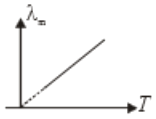2.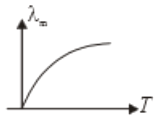3.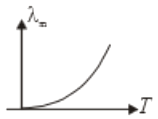4.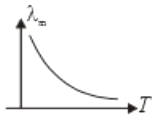Complete Question Bank + Test Series
Complete Question Bank

Difficulty Level:

If 48 gm of ${\mathrm{O}}_{3}$ at $27°\mathrm{C}$ is mixed with 64 gm of ${\mathrm{O}}_{2}$ at $327°\mathrm{C}$ in an adiabatic vessel as shown in the figure, then the final temperature of the mixture will be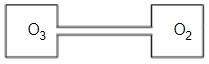1.  $200°\mathrm{C}$

2.  $227°\mathrm{C}$

3.  $314.5°\mathrm{C}$

4.  $235.5°\mathrm{C}$

Complete Question Bank + Test Series
Complete Question Bank

Difficulty Level:

Heat capacity is equal to the product of:

1.  mass and gas constant

2.  mass and specific heat

3.  latent heat and volume of water